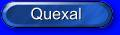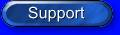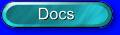# SSE Reciprocal instructionsA basic building block operation in geometry involves computing divisions and square roots. For instance, transformation often involves dividing each x, y, z coordinate by the W perspective coordinate; normalization is another common geometry operation, which requires the computation of 1/square-root. In order to optimize these cases, SSE introduces two approximation instructions: RCP and RSQRT. These instructions are implemented via hardware lookup tables and are inherently less precise (12 bits of mantissa) than the full IEEE-compliant DIV and SQRT (24 bits of mantissa), but have the advantage of being much faster than the full precision versions. When greater precision is needed, the approximation instructions can be used with a single Newton-Raphson iteration to achieve almost the same precision as the IEEE instructions (~22 bits of mantissa). For a basic geometry pipeline, these instructions can improve overall performance on the order of 15%.

 RCPSS xmm1, xmm2/m32 Computes of an approximate reciprocal of the low single-precision floating-point value in the source operand (second operand) stores the single-precision floating-point result in the destination operand. The source operand can be an XMM register or a 32-bit memory location. The destination operand is an XMM register. The three high-order doublewords of the destination operand remain unchanged.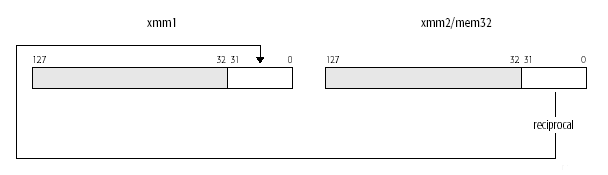DEST[31-0] ← APPROX (1.0/(SRC[31-0])); RCPSS __m128 _mm_rcp_ss(__m128 a)

 RCPPS xmm1, xmm2/m128 Performs a SIMD computation of the approximate reciprocals of the four packed single-precision floating-point values in the source operand (second operand) stores the packed single-precision floating-point results in the destination operand. The source operand can be an XMM register or a 128-bit memory location. The destination operand is an XMM register.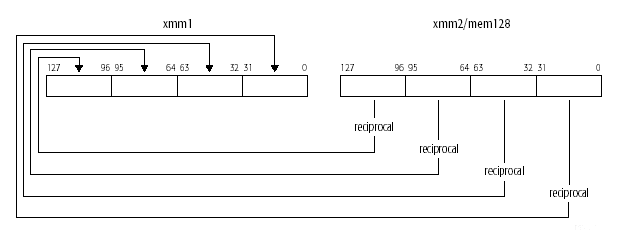DEST[31-0] ← APPROXIMATE(1.0/(SRC[31-0])); DEST[63-32] ← APPROXIMATE(1.0/(SRC[63-32])); DEST[95-64] ← APPROXIMATE(1.0/(SRC[95-64])); DEST[127-96] ← APPROXIMATE(1.0/(SRC[127-96])); RCCPS __m128 _mm_rcp_ps(__m128 a)

 RSQRTSS xmm1, xmm2/m32 Computes an approximate reciprocal of the square root of the low single-precision floating point value in the source operand (second operand) stores the single-precision floating-point result in the destination operand. The source operand can be an XMM register or a 32-bit memory location. The destination operand is an XMM register. The three high-order doublewords of the destination operand remain unchanged.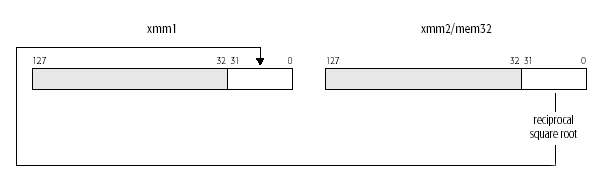DEST[31-0] ← APPROXIMATE(1.0/SQRT(SRC[31-0])); RSQRTSS __m128 _mm_rsqrt_ss(__m128 a)

 RSQRTPS xmm1, xmm2/m128 Performs a SIMD computation of the approximate reciprocals of the square roots of the four packed single-precision floating-point values in the source operand (second operand) and stores the packed single-precision floating-point results in the destination operand. The source operand can be an XMM register or a 128-bit memory location. The destination operand is an XMM register.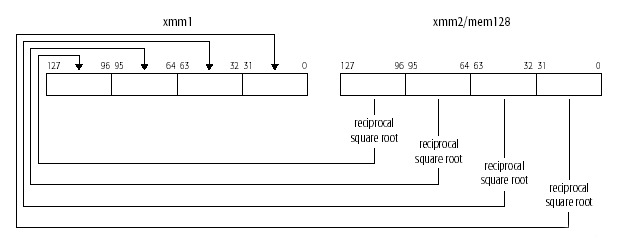DEST[31-0] ← APPROXIMATE(1.0/SQRT(SRC[31-0])); DEST[63-32] ← APPROXIMATE(1.0/SQRT(SRC[63-32])); DEST[95-64] ← APPROXIMATE(1.0/SQRT(SRC[95-64])); DEST[127-96] ← APPROXIMATE(1.0/SQRT(SRC[127-96])); RSQRTPS __m128 _mm_rsqrt_ps(__m128 a)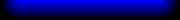Copyright Stefano Tommesani 2000/03 - All trademarks belong to their respective holders